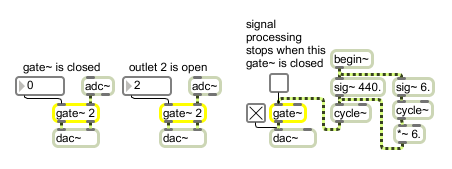# gate~

Route a signal to one of several outlets

## Description

gate~ is a version of the Max gate object designed for signals. It takes an argument for number-of-outputs (one is the default) and lets you route an incoming signal by sending an int to its left inlet. If there is more than one input, gate~ shuts off all outputs except the one you select, where 1 selects the left outlet, 2 selects the next one to the right, etc. gate~ can also control the activation or deactivation of part of a signal processing network. For an example, see the begin~ help file. Note: "shutting off" a signal means disconnecting it from a gate~ output and outputting a zero signal instead. This is different from the behavior of the Max gate object.

## Arguments

Name Type Opt Description
number-of-outlets int opt The first argument specifies the number of outlets. The default is 1. The second argument sets the outlet that will initially send out the input signal. The default is 0, where all signals are shut off and zero signals are sent out all outlets. If a signal is connected to the left inlet, the second argument is ignored.

## Messages

 int outlet-selected [int] In left inlet: Determines the outlet that will send out the signal coming in the right inlet. If the number is 0 or negative, the right inlet is shut off and a zero signal is sent out. If the number is greater than the number of outlets, the signal is sent out the rightmost outlet. If a signal is connected to the left inlet, gate~ ignores int or float messages received in its left inlet. signal In left inlet: If a signal is connected to the left inlet, gate~ operates in a mode that uses signal values to determine the outlet that will receive its input signal (the signal coming in the right inlet). If the signal coming in the left inlet is 0 or negative, the inlet is shut off and a zero signal is sent out. If it is greater than or equal to 1, but less than 2, the input signal goes to the left outlet. If the signal is greater than or equal to 2 but less than 3, the input signal goes to the next outlet to the right, and so on. If the signal in the left inlet is greater than the number of outlets, the rightmost outlet is used. In right inlet: The input signal to be passed through to one of the gate~ object's outlets, according to the most recently received int or float in the left inlet, or the value of the signal coming in the left inlet. If the signal network connected to the right inlet of gate~ contains a begin~ object -- and a signal is not connected to the left inlet of the gate~-- all processing between the begin~ outlet and the gate~ inlet will be turned off when gate~ is shut off.

## Output

signal: Depending on the value of the left inlet (either signal or number), one of the object's outlets will send out the input signal and rest will send out zero signals, or (if the inlet is closed) all outlets will send out zero signals.

## Examplesgate~ routes the input signal to one of its outlets or shuts it off entirely